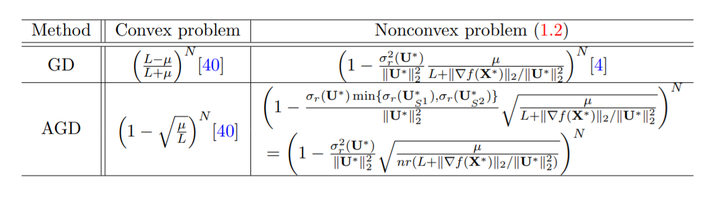# Provable Accelerated Gradient Method for Nonconvex Low Rank Optimization### Abstract

Optimization over low rank matrices has broad applications in machine learning. For large scale problems, an attractive heuristic is to factorize the low rank matrix to a product of two much smaller matrices. In this paper, we study the nonconvex problem minU∈Rn×r g(U) = f(UUT) under the assumptions that f(X) is restricted µ-strongly convex and L-smooth on the set {X : X \geq 0,rank(X) ≤ r}. We propose an accelerated gradient method with alternating constraint that operates directly on the U factors and show that the method has local linear convergence rate with the optimal dependence on the condition number of p L/µ. Globally, our method converges to the critical point with zero gradient from any initializer. Our method also applies to the problem with the asymmetric factorization of X = Ue Ve T and the same convergence result can be obtained. Extensive experimental results verify the advantage of our method.

Type
Publication
Machine Learning